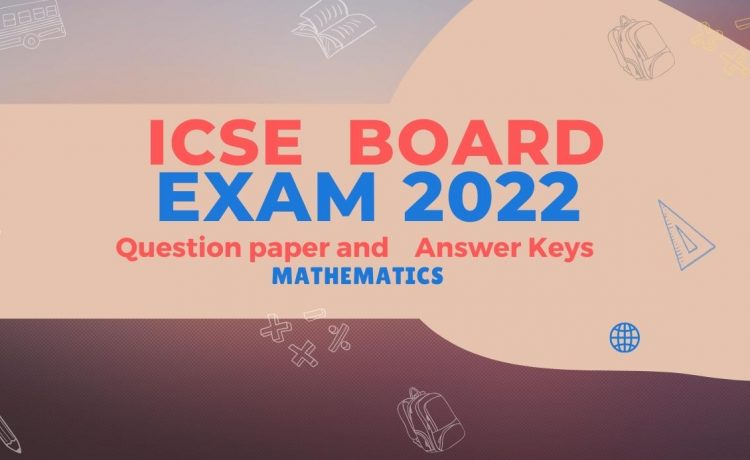# ICSE STD 10 Board Exam 2022-MATHS Question Paper and KeyDownload ICSE Board exam 2022 question paper and solutions of Mathematics. The Semester 2 examination of The Indian Certificate of Secondary Education (ICSE) students. The Maths examination was held on 3rd May 2022 Tuesday.

## Sample questions from ICSE Maths Paper 2022

1. The probability of getting a number divisible by 3 in throwing a dice is :
(a)  1/6       (b)  1/3         (c)   1/2         (d)   2/3

2. The volume of a conical tent is 462 m3 and the area of the base is 154 m². The height of the cone is :
(a) 15 m                (b) 12 m
(c) 9 m                 (d) 24 m

3. The coordinates of the point  P(-3, 5) on reflecting on the x axis are :
(a)   (3,5)           (b)   (-3,-5)
(c)  (3,-5)           (d)   (-3,5)

4. Find ‘a’, if A(2a+2,3), B(7,4) and C(2a + 5,2) are collinear.

5. A solid cone of radius 5 cm and height 9 cm is melted and made into small cylinders of radius of 0.5 cm and height 1.5 cm. Find the number of cylinders so formed.

6. Two lamp posts AB and CD each of height 100 m are on either side of the road. P is a point on the road between the two lamp posts. The angles of elevation of the top of the lamp posts from the point P are 60° and 40°. Find the distances PB and PD.

7.  A bag contains 5 white, 2 red and 3 Mark balls. A ball is drawn at random. What is the probability that the ball drawn in a red ball?

8. A solid wooden cylinder is of radius 6 cm and height 16 cm. Two cones each of radius 2 cm and height 6 cm are drilled out of the cylinder. Find the volume of the remaining solid.

9. Find the equation of a line parallel to the line 2x + y – 7 = 0 and passing through the intersection of the lines x + y – 4 = 0 and 2x – y = 8.

10. Two chords AB and CD of a  circle. Intersect externally at E. If EC = 2 cm, EA = 3 cm and AB = 5 cm, find the length of CD.

ICSE 2022 Maths Solved Paper PDF

## Related Pages

•Sera Mariam says:
•Sahithi says:
•Rijesh says: